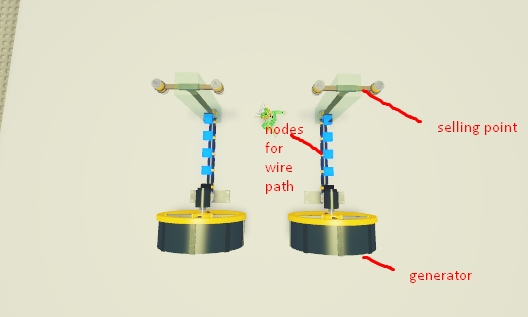# How can I optimize this?

In my game, I have a wire system where a generator produces energy at a certain rate, sends it along a path, and the seller turns the energy into money.However, every time energy is sent it creates a new thread where it iterates over the path, sends energy to the next node in the path, and then yields

The problem with this is that it quickly creates 100+ threads very very quickly, and I also plan to increase the speed on future generator items in the game later, which will certainly raise that thread count even higher.

Is there something I’m potentially missing that I can use to mitigate the number of threads with this?

I would make an array of ‘energy’ objects, they would have a reference to the next node in the path. And a single thread iterates through the array of energy objects and updates them. It’s like an array of iterators in the queue and a thread updates them all at a time.

So basically every generator uses that one array and inserts these objects into it, then the other thread, mentioned above, makes them move along their paths.

1 Like

Why should he/she not just make everything part of a node system? The generator and selling points can be considered nodes, if the entire node system is considered connected then it will generate cash. This would also make it not require making many threads.

1 Like

The game sorta has a mechanic that relies on each wire having a energy value and the wire sending out energy at a certain speed. Currently every single item is considered part of the node system (as long as it’s attached to a generator as that’s the starting point for where the nodes generate)

That’s sounds even better, generators and selling points also should be nodes in the system.

So the nodes I mentioned can store the value and in a loop the energy would be sent to the next node. And the rate at which the energy is being passed can be solved as following: every node will be updated at the same rate but some of them will pass more energy to the next node and some of them (the ones that send energy slower) will pass less energy and all this in a single ‘tick’ of your system.

1 Like

What you’re looking for is an ordered tree. Create a node class that contains a energy value and a table of child nodes, could have only one value if it’s a direct connection or multiple if the wire splits off. The first node starts at the generator, and the last nodes will be at the devices being powered by it.

Once you’ve created the node tree, you set the value of the first node to max and iterate over each sub-node in the first node’s “childNodes” table. You then wait a specified amount of time, and repeat the process starting with each sub-node. You’d only need one thread per generator this way.

EDIT: To compensate for energy / speed differences you can set a “letThrough” value in each node which is the amount of energy it’ll pass per tick, for example, if the generator generates 50 energy per tick and the node has a “letThrough” of 25, it’ll only pass along 25 energy. You can add a function to check if the stored energy passes the max energy limit (could be set to 50) thus it’d take two ticks in this case to progress to the next node. The other nodes would be unaffected because you walk through the tree by index(or children) instead of yielding, the only thing that’s yielding the thread would be the ticker.

2 Likes

I wrote a little script for reference, I wrote this from my head and didn’t test or double-check it, but it should guide you in the right direction.

``````local GENERATOR_ENERGY_OUTPUT = 50
local CAN_DEPLETE = true

-- Node
local Node = {}
Node.__index = Node

Node.new = function()
local instance = {}
instance.maxEnergy = 50
instance.storedEnergy = 0
instance.letThrough = 25
instance.children = nil

return setmetatable(instance, Node)
end

Node.CanTick = function(self)
return self.storedEnergy >= self.maxEnergy
end

Node.OnTick = function(self, energy)
local addedEnergy = energy >= self.letThrough and self.letThrough or energy

if self.storedEnergy > self.maxEnergy then
self.storedEnergy = self.maxEnergy
else
if CAN_DEPLETE then
if self.storedEnergy ~= self.maxEnergy then
end
end
end

if self:CanTick() and energy > 0 then
for _, child in pairs(self.children) do
child:OnTick(energy)
end
end
end
-- Node End

local generatorNodes = {}

coroutine.wrap(function()
while true do
for _, node in pairs(generatorNodes) do
node:OnTick(GENERATOR_ENERGY_OUTPUT)
end

wait(TICK_DELAY)
end
end)()``````
1 Like